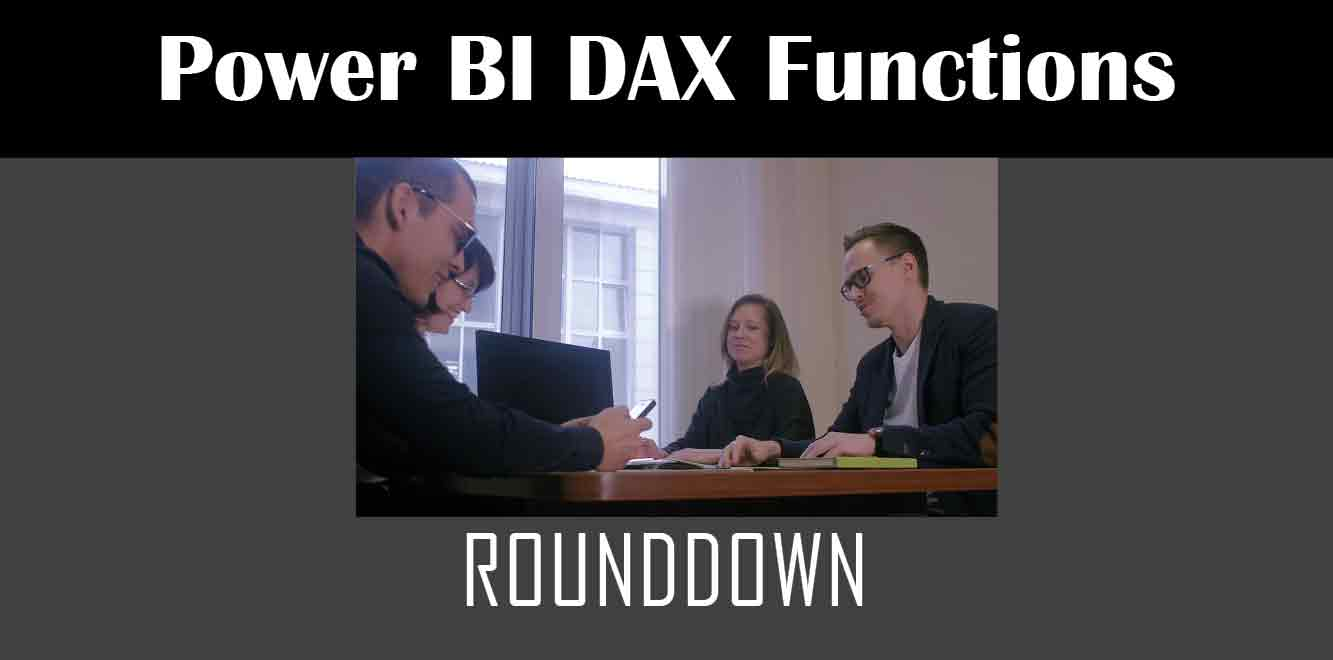# RoundDown

## Y## What is the RoundDown Function?

The RoundDown function in Power Apps is used to round a number down to a specified number of decimal places. For example, if you have a number like 3.14159 and you want to round it down to two decimal places, the RoundDown function will return 3.14.

The syntax for the RoundDown function is as follows:

RoundDown(number, num_digits)

``` Where number is the number you want to round down and num_digits is the number of decimal places you want to round down to. How to Use the RoundDown Function in Power Apps Using the RoundDown function in Power Apps is easy. You can use it in any formula where you need to round a number down to a specified number of decimal places. Here is an example: Suppose you have a number field in your Power App called "Price". You want to calculate the total price of an order by multiplying the price by the quantity and then rounding the result down to two decimal places. You can use the RoundDown function in the following formula: ```

RoundDown(Price * Quantity, 2)

``` This formula will multiply the value in the "Price" field by the value in the "Quantity" field, and then round the result down to two decimal places. Other Useful Functions for Rounding Numbers in Power Apps In addition to the RoundDown function, Power Apps also provides other useful functions for rounding numbers, including: - **RoundUp:** This function rounds a number up to a specified number of decimal places. - **Round:** This function rounds a number to a specified number of decimal places, with the option to round up or down based on the value of the next digit. - **Floor:** This function rounds a number down to the nearest integer. - **Ceiling:** This function rounds a number up to the nearest integer. The RoundDown function is a useful tool for rounding numbers down to a specified number of decimal places in Power Apps. By using this function in your formulas, you can ensure that your calculations are accurate and consistent. Additionally, Power Apps provides several other useful functions for rounding numbers, which you can use to further customize your applications. Power Apps Training Courses by G Com Solutions (0800 998 9248)1-Week Intensive Power Apps Training Course £4,975.00 Select optionsContinue Loading DonePower Apps Introduction (2 Days) £1,990.00 Select optionsContinue Loading DonePower Apps Intermediate (1 Day) £995.00 Select optionsContinue Loading DonePower Apps Advanced (2 Days) £1,990.00 Select optionsContinue Loading Done ```
``` ```
``` ```
``` ```
``` ```
``` ```